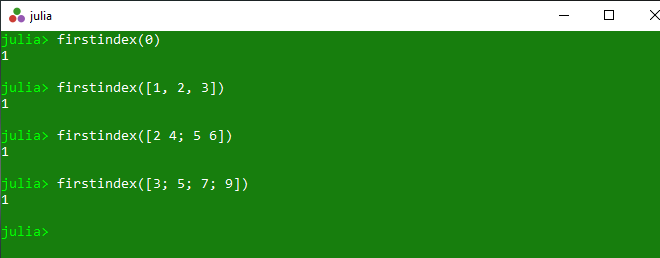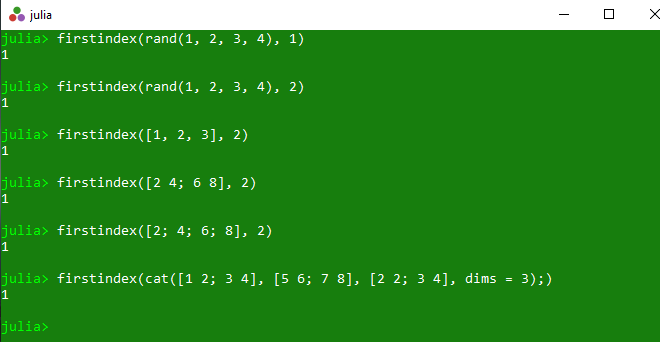# Getting first index of a collection in Julia – firstindex() Methods

The `firstindex()` is an inbuilt function in julia which is used to return the first index of the specified collection. If a dimension d is given, return the first index of collection along dimension d.

Syntax:
firstindex(collection)
or
firstindex(collection, d)

Parameters:

• collection: Specified collection.
• b: Specified dimension.

Returns: It returns the first index of the specified collection. If a dimension d is given, return the first index of collection along dimension d.

Example 1:

 `# Julia program to illustrate  ` `# the use of firstindex() method ` `  `  `# Getting the first index of the specified collection. ` `println(firstindex(``0``)) ` `println(firstindex([``1``, ``2``, ``3``])) ` `println(firstindex([``2` `4``; ``5` `6``])) ` `println(firstindex([``3``; ``5``; ``7``; ``9``])) `

Output:Example 2:

 `# Julia program to illustrate  ` `# the use of firstindex() method ` `  `  `# Getting the first index of the specified  ` `# collection. If a dimension d is given,  ` `# return the first index of collection  ` `# along dimension d. ` `println(firstindex(rand(``1``, ``2``, ``3``, ``4``), ``1``)) ` `println(firstindex(rand(``1``, ``2``, ``3``, ``4``), ``2``)) ` `println(firstindex([``1``, ``2``, ``3``], ``2``)) ` `println(firstindex([``2` `4``; ``6` `8``], ``2``)) ` `println(firstindex([``2``; ``4``; ``6``; ``8``], ``2``)) ` `println(firstindex(cat([``1` `2``; ``3` `4``], [``5` `6``; ``7` `8``], [``2` `2``; ``3` `4``], dims ``=` `3``);)) `

Output:Whether you're preparing for your first job interview or aiming to upskill in this ever-evolving tech landscape, GeeksforGeeks Courses are your key to success. We provide top-quality content at affordable prices, all geared towards accelerating your growth in a time-bound manner. Join the millions we've already empowered, and we're here to do the same for you. Don't miss out - check it out now!

Previous
Next